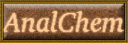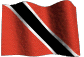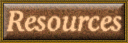# Parts Per Million Conversions

PPM conversion values and serial dilutions :
Ppm concentrations and percentages.
Ppm to Molarity and Molarity to ppm.

HOME Email Webmaster

1000ppm AA Stds.

### PPM = parts per million

PPM is a term used in chemistry to denote a very, very low concentration of a solution. One gram in 1000 ml is 1000 ppm and one thousandth of a gram (0.001g) in 1000 ml is one ppm.

One thousanth of a gram is one milligram and 1000 ml is one liter, so that 1 ppm = 1 mg per liter = mg/Liter.
PPM is derived from the fact that the density of water is taken as 1kg/L = 1,000,000 mg/L, and 1mg/L is 1mg/1,000,000mg or one part in one million.

### OBSERVE THE FOLLOWING UNITS

1 ppm = 1mg/l = 1ug /ml = 1000ug/L
ppm = ug/g =ug/ml = ng/mg = pg/ug = 10 -6
ppm = mg/litres of water

1 gram pure element disolved in 1000ml = 1000 ppm

PPB = Parts per billion = ug/L = ng/g = ng/ml = pg/mg = 10 -9

### Making up 1000 ppm solutions

1. From the pure metal : weigh out accurately 1.000g of metal, dissolve in 1 : 1 conc. nitric or hydrochloric acid, and make up to the mark in 1 liter volume deionised water.

2. From a salt of the metal :
e.g. Make a 1000 ppm standard of Na using the salt NaCl.

FW of salt = 58.44g.
At. wt. of Na = 23
1g Na in relation to FW of salt = 58.44 / 23 = 2.542g.
Hence, weigh out 2.542g NaCl and dissolve in 1 liter volume to make a 1000 ppm Na standard.

3. From an acidic radical of the salt :
e.g. Make a 1000 ppm phosphate standard using the salt KH2PO4

FW of salt = 136.09
FW of radical PO4 = 95
1g PO4 in relation to FW of salt = 136.09 / 95 = 1.432g.
Hence, weigh out 1.432g KH2PO4 and dissolve in 1 liter volume to make a 1000 ppm PO4 standard.
Click this link for Atomic absorption standards

### Dilution Formula : C1V1 = C2V2

This equation applies to all dilution problems.

C1 (initial conc) x V1 (initial volume) = C2 (final conc) x V2 (final volume)

Example : What volume of 6.00 ppm solution must be used to give 4.00 liters of a 0.100 ppm solution?

C1 = 6.00 ppm
V1 = unknown
C2 = 0.100 ppm
V2 = 4 liters = 4000 mls

V1 = (C2 x V2) / C1

= (0.100 X 4000) / 6.00
= 400 / 6.00 = 66.7 mls.

This means that 66.7 mls of the 6.00ppm solution diluted to a final volume of 4 liters will give a concentration of 0.100 ppm.

The Formula below can be used to calculate the the V1 component only.

req is the value you want.

req ppm x req vol
-------------------------- = no of mls for req vol
stock

Example : Make up 50 mls vol of 25 ppm from 100 ppm standard.

25 x 50 / 100 = 12.5 mls. i.e. 12.5 mls of 100 ppm in 50 ml volume will give a 25 ppm solution

### Serial dilutions

Making up 10-1 M to 10-5 M solutions from a 1M stock solution.

Pipette 10 ml of the 1M stock into a 100 ml volumetric flask and make up to the mark to give a 10-1 M soln.
Now, pipette 10 ml of this 10-1 M soln. into another 100 ml flask and make up to the mark to give a 10-2 M soln.
Pipette again, 10 ml of this 10-2 M soln. into yet another 100 ml flask and make up to mark to give a 10-3 M soln.
Pipette a 10 ml of this 10-3 M soln. into another 100 ml flask and make up to mark to give a 10-4 M soln.
And from this 10-4 M soln. pipette 10 ml into a 100 ml flask and make up to mark to give a final 10-5 M solution.

### Molarity to ppm

Convert molar concentration to grams per liter (Molarity x Atomic mass of solute), then convert to milligrams per liter (ppm) by multiplying by 1000.

e.g. What is the ppm concentration of calcium ion in 0.01M CaCO3?

Molarity(M) x Atomic mass(At Wt) = grams per liter(g/l)
Atomic Mass (Wt.) of Ca = 40

0.01M x 40 =0.40 g/l
0.40g/l x 1000 = 400 mg/l = 400ppm

Note:
The FW of an ion species is equal to its concentration in ppm at 10-3M. Fluoride has a FW of 19, hence a 10-3M concentration is equal to 19ppm, 1M is equal to 19,000 ppm and 1ppm is equal to 5.2 x 10-5M.
Go here for
ISE molarity/ppm conversions shown in Table III.

### PPM to Molarity

Convert ppm to gram based or milligram based concentration.

ppm = 1 mg solute per liter solution or
ppm = 0.001 gram per liter solution

e.g. What is the Molarity of 400ppm Ca ions in an aqueous CaCO3 solution?

Using the 0.001g/l concentration: 400ppm x 0.001g/l = 0.4g/l.
or, Divide 400 mg by 1000 to get g/l = 0.4 g/l
Now divide by the At. Mass of Ca to get Molarity.
0.4g/l divided by 40g/mol =0.01M

Using the mg/l concentration, the 40g Ca must be converted to milligrams by multiplying by 1000 to give 40,000mg.
Hence Molarity = 400ppm divided by 40,000mg/mol = 0.01M

### Ppm (parts per million) to % (parts per hundred)

Divide the ppm amount by 1,000,000 and multiply by 100 to get %. e.g. :

1 ppm = 1/1,000,000 = 0.000001 = 0.0001%
10 ppm = 10/1,000,000 = 0.00001 = 0.001%
100 ppm = 100/1,000,000 = 0.0001 = 0.01%
200 ppn = 200/1,000,000 = 0.0002 = 0.02%
5000 ppm = 5000/1,000,000 = 0.005 = 0.5%
10,000 ppm = 10000/1,000,000 = 0.01 = 1.0%
20,000 ppm = 20000/1,000,000 = 0.02 = 2.0%

### (Parts per hundred) % to ppm

Divide the % value by 100 and multiply by 1,000,000 to get ppm. e.g. :

1% =0.01 x 1,000,000 = 10,000 ppm
0.5% =0.0.005 x 1,000,000 = 5,000 ppm
0.1% =0.001 x 1,000,000 = 1,000 ppm
0.01% = 0.0001 x 1,000,000 = 100 ppm

delloyd.50megs.com

Signature: Dhanlal De Lloyd, Chem. Dept, The University of The West Indies, St. Augustine campus
The Republic of Trinidad and Tobago.